# How to approach a Machine Learning project : A step-wise guidance

This article will provide a basic procedure on how should a beginner approach a Machine Learning project and describe the fundamental steps involved. In the problem, we will focus on the classification of iris flowers. You can learn about the dataset here.

Many teachers and websites take up this problem to demonstrate the various nuances involved in a Machine Learning project because –

1. All the attributes are numeric and all the attributes are of same scale and units.
2. The problem in hand is a classification problem and thus gives us an option to explore many evaluation metrics.
3. The dataset involved is a small and clean and thus can be handled easily.

We demonstrate the following steps and describe them accordingly along the way.

Step 1: Importing the required libraries

 `import` `pandas as pd ` `from` `pandas.plotting ``import` `scatter_matrix ` `import` `matplotlib.pyplot as plt ` `from` `sklearn ``import` `model_selection ` `from` `sklearn.metrics ``import` `classification_report, confusion_matrix, accuracy_score ` `from` `sklearn.linear_model ``import` `LogisticRegression ` `from` `sklearn.tree ``import` `DecisionTreeClassifier ` `from` `sklearn.neighbors ``import` `KNeighborsClassifier `

 `# dataset (csv file) path ` `url ``=` `"https://raw.githubusercontent.com /jbrownlee/Datasets/master/iris.csv"` ` `  `# selectng necessary feature ` `features ``=` `[``'sepal-length'``, ``'sepal-width'``, ``'petal-length'``, ``'petal-width'``, ``'class'``] ` ` `  `# reading the csv ` `data ``=` `pd.read_csv(url, names ``=` `features) `

Step 3: Summarizing the Data

This step typically involves the following steps-

a) Taking a peek at the Data

 `data.head() `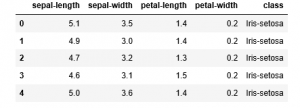b) Finding the dimensions of the Data

 `data.shape `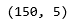c) Statistical summary all attributes

 `print``(data.describe()) `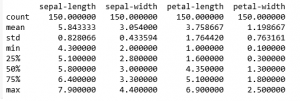d) Class distribution of the Data

 `print``((data.groupby(``'class'``)).size()) `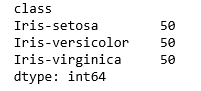Step 4: Visualising the Data

This step typically involves the following steps –

a) Plotting Univariate plots

This is done to understand the nature of each attribute.

 `data.plot(kind ``=``'box'``, subplots ``=` `True``, layout ``=``(``2``, ``2``),  ` `                       ``sharex ``=` `False``, sharey ``=` `False``) ` ` `  `plt.show() `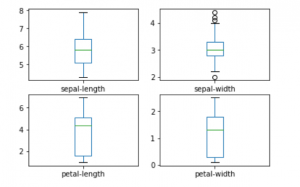`data.hist() ` `plt.show() `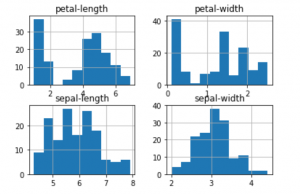b) Plotting Multivariate plots

This is done to understand the relationships between different features.

 `scatter_matrix(data) ` `plt.show() `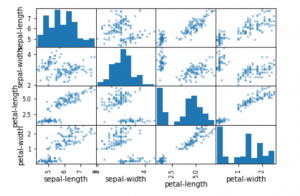Step 5: Training and Evaluating our models

This step typically contains the following steps –

a) Splitting the training and testing data

This is done so that some part of the data is hidden from the learning algorithm

 `y ``=` `data[``'class'``] ` `X ``=` `data.drop(``'class'``, axis ``=` `1``) ` `X_train, X_test, y_train, y_test ``=` `model_selection.train_test_split( ` `                           ``X, y, test_size ``=` `0.25``, random_state ``=` `0``) ` ` `  `print``(X.head()) ` `print``('') ` `print``(y.head()) `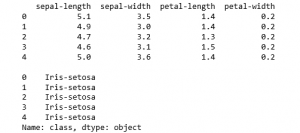b) Building and Cross-Validating the model

 `algorithms ``=` `[] ` `scores ``=` `[] ` `names ``=` `[] ` ` `  `algorithms.append((``'Logisitic Regression'``, LogisticRegression())) ` `algorithms.append((``'K-Nearest Neighbours'``, KNeighborsClassifier())) ` `algorithms.append((``'Decision Tree Classifier'``, DecisionTreeClassifier())) ` ` `  `for` `name, algo ``in` `algorithms: ` `    ``k_fold ``=` `model_selection.KFold(n_splits ``=` `10``, random_state ``=` `0``) ` ` `  `    ``# Applying k-cross validation ` `    ``cvResults ``=` `model_selection.cross_val_score(algo, X_train, y_train, ` `                                      ``cv ``=` `k_fold, scoring ``=``'accuracy'``) ` ` `  `    ``scores.append(cvResults) ` `    ``names.append(name) ` `    ``print``(``str``(name)``+``' : '``+``str``(cvResults.mean())) `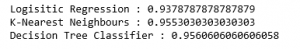c) Visually comparing the results of the different algorithms

 `fig ``=` `plt.figure() ` `fig.suptitle(``'Algorithm Comparison'``) ` `ax ``=` `fig.add_subplot(``111``) ` `plt.boxplot(scores) ` `ax.set_xticklabels(names) ` `plt.show() `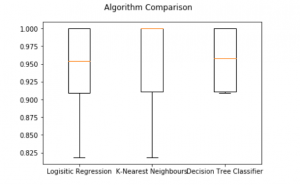Step 6: Making predictions and evaluating the predicitons

 `for` `name, algo ``in` `algorithms: ` `    ``clf ``=` `algo ` `    ``clf.fit(X_train, y_train) ` `    ``y_pred ``=` `clf.predict(X_test) ` `    ``pred_score ``=` `accuracy_score(y_test, y_pred) ` ` `  `    ``print``(``str``(name)``+``' : '``+``str``(pred_score)) ` `    ``print``('') ` `    ``print``(``'Confusion Matrix: '``+``str``(confusion_matrix(y_test, y_pred))) ` `    ``print``(classification_report(y_test, y_pred)) `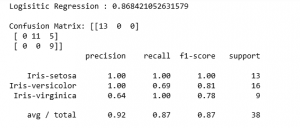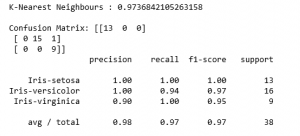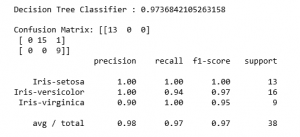My Personal Notes arrow_drop_upCheck out this Author's contributed articles.

If you like GeeksforGeeks and would like to contribute, you can also write an article using contribute.geeksforgeeks.org or mail your article to contribute@geeksforgeeks.org. See your article appearing on the GeeksforGeeks main page and help other Geeks.

Please Improve this article if you find anything incorrect by clicking on the "Improve Article" button below.

Article Tags :
Practice Tags :

4

Please write to us at contribute@geeksforgeeks.org to report any issue with the above content.